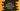# lgamma function of Python math## lgamma :

lgamma function is defined in python math module. It is used to find the natural logarithm of the absolute value of the gamma function for an argument. This function is available for python 3.2 and above.

### Syntax and parameters :

Below is the syntax of lgamma :

``Math.lgamma(x)``

x can be a number or valid numerical expression.

• The argument can be positive integer, positive or negative decimal.
• It can throw TypeError or ValueError. If the argument is not a number, it throws TypeError and if it is a negative integer, it throws ValueError.

### Return value :

lgamma returns the natural logarithm of the absolute value of the gamma function for x.

### Example of python lgamma :

Let’s try python lgamma with different arguments :

``````import math

values = [10, 10.0, 24.5, -23.5, 0.0, 0, -0.0, "Hello", -10]

for i in values :
try:
print("{} : {}".format(i,math.lgamma(i)))
except TypeError:
print("TypeError for {}".format(i))
except ValueError:
print("ValueError for {}".format(i))``````

Here, we are using an array values of different types of arguments. The for..in loop runs through the array elements one by one and uses math.lgamma on each. We are executing lgamma in a try-catch block and printing out TypeError and ValueError.

It will print the below output :

``````10 : 12.801827480081467
10.0 : 12.801827480081467
24.5 : 53.19049452616927
-23.5 : -52.04576464031987
ValueError for 0.0
ValueError for 0
ValueError for -0.0
TypeError for Hello
ValueError for -10``````

It throws ValueError for negative integer and 0. For any other format other than number, it throws TypeError.